# Conversion centiliters to U.S.s gallon, cl to gal.

The conversion factor is 0.0026417205235815; so 1 centiliter = 0.0026417205235815 U.S.s gallon. In other words, the value in cl divide by 378.5411784 to get a value in gal. The calculator gives the answer to the questions: 120 cl is how many gal? or change cl to gal. Convert cl to gal.

## Conversion result:

### 1 cl = 0.002642 gal

1 centiliter is 0.002642 U.S.s gallon.

cl
Essential of conversions SI units of the volume is the coefficient 1000. Units of volume are the cubes of units of length. For example, 1 dm3 = 103 cm3 = 1000 cm3. Another important rule is definition 1 liter = 1 dm3. Imperial volume units use nontrivial coefficients for conversions. ie. 1 gal = 3.78541 liters

### Conversion of a volume unit in word math problems and questions

• Excavation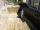Excavation for the base of the cottage has dimensions 4.5 m x 3.24 m x 60 cm. The excavated soil will increase its volume by one-quarter. Calculate the volume of excavated soil.
• Cooling liquid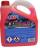Cooling liquid is diluted with water in a ratio of 3:2 (3 parts by volume of coolant with 2 volumes of water). How many coolant volumes must be prepared for a total of 0.7 dm3 (liters) of the mixture?
• Cube and waterHow many liters of water can fit into a cube with an edge length of 0.11 m?
• Pumps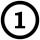First pump flows 16 liters per second into the basin by the second pump 75% of the first and by third pump half more than the second. How long will take fill basin by all three pumps simultaneously volume 15 m3 (cubic meters)?
• ContainerThe container-shaped box with internal dimensions of 3.9 m, 3.25 m, and 2.6 m was completely filled with goods in the same cubic boxes. How long edge could this box have?
• Copper plateCalculate the thickness of the copper plate with a density 8.7 g/cm³ measuring 1.5 meters and 80 cm and its weight is 3.65 kg
• Tetrahedral prism - rhomboid baseCalculate the area and volume tetrahedral prism that has base rhomboid shape and its dimensions are: a = 12 cm, b = 70 mm, v_a = 6 cm, v_h = 1 dm.
• FreezerThe freezer has the shape of a cuboid with internal dimensions of 12 cm, 10 cm, 30 cm. A layer of ice of 23 mm thick was formed on the inner walls (and on the opening) of the freezer. How many liters of water will drain if we dispose the freezer?
• Tetrahedral pyramidCalculate the volume and surface area of a regular tetrahedral pyramid, its height is \$b cm and the length of the edges of the base is 6 cm.
• Hectoliters of waterThere are 942 hectoliters of water in a cylindrical tank with an inner diameter of 6 m. The water reaches two thirds of the depth of the tank. Calculate its depth.
• Tank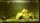The tank bottom has dimensions of 1.5 m and 3 2/6 m. The tank is 459.1 hl of water. How high is the water surface?
• TrayWhat height reach water level in the tray shaped a cuboid, if it is 420 liters of water and bottom dimensions are 120 cm and 70 cm.
• Swimming poolThe swimming pool has the shape of a block with dimensions of 70dm, 25m, 200cm. How many hl of water can fit into the pool?
• Runcated pyramid teapot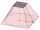The 35 cm high teapot has the shape of a truncated pyramid with the length of the edge of the lower square base a=50 cm and with the edges of the rectangular base b: 20 cm and c: 30 cm. How many liters of water will fit in the teapot?
• Snails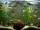How many liters of water will fit in an aquarium with bottom dimensions of 30 cm and 25 cm and a height of 60 cm, if we pour water up to a height of 58 cm? How many most snails can we keep in an aquarium, if we know that snails need 600 cm ^ 3 of water fo